﻿ 化学键的量子化学研究(三) A Quantum Chemistry Study of the Chemical Bond (3)

Journal of Advances in Physical Chemistry
Vol.07 No.03(2018), Article ID:26323,10 pages
10.12677/JAPC.2018.73015

A Quantum Chemistry Study of the Chemical Bond (3)

Guangyao Zhou

Beijing Kein Research Center for Natural Sciences, Beijing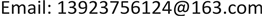Received: Jul. 20th, 2018; accepted: Aug. 2nd, 2018; published: Aug. 9th, 2018ABSTRACT

Through quantum chemical calculations, molecular orbital and electron density difference analyses were performed. From the perspective of the Hellman-Feynman electrostatic theorem, multi-center bond is strictly defined. Multi-center bonds are also the binding forces caused by the sharing of electrons between atoms. Some formal multi-center bonds are discussed.

Keywords:Chemical Bond, Electron Sharing, Multi-Center Bond, σ-Hyper Conjugative Effect1. 前言

2. 多中心键的定义

2.1. 多中心键的定义

${\text{H}}_{{}_{\text{3}}}^{+}$ 是一个公认的三中心二电子(3c2e)键  ，此外没有任何其它的键，其实，从 ${\text{H}}_{{}_{\text{3}}}^{+}$ 就可以抽象归纳出多中心键定义和特性。它天生就是可以用来定义多中心键的一个客观实体。

${\text{H}}_{{}_{\text{3}}}^{+}$ 并结合着其它多中心键，可以抽象出多中心键的定义和性质如下：

2.2. 多中心键与 $\Delta \rho$

${\text{H}}_{{}_{\text{3}}}^{+}$ ，用ωB97XD方法  进行计算，并作相关的 $\Delta \rho$ 图像(自由H原子wfn的电子占据取0.6666667)。这时原子所围成势场中央空间的 $\Delta \rho$ 呈现聚集密度增加的状态，并且从电子密度增加的情形可以看出多中心键构成的脉络、路径。图1(A)就是 ${\text{H}}_{{}_{\text{3}}}^{+}$ 共享电子在原子势场中央密度增加的情形。

AdNDP (Adaptive natural density partitioning适应性自然密度划分)  是专门用来搜索分子体系多中心轨道的程序，已经集成在Multiwfn之中了，它用穷举法找到分子中所有可能的各种多中心轨道作为候选轨道列表，从中可能找到多中心键。图1(C)就是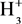由AdNDP作的三中心键的电子占据情形，电子密度高处在3中心的中央，等值面取值0.2，它展示了这个三中心键共享电子中央成键区的大致区划。

3. 多中心键的一些实例

3.1. $L{i}_{5}^{+}$ 团簇

3.2. 二硼烷B2H6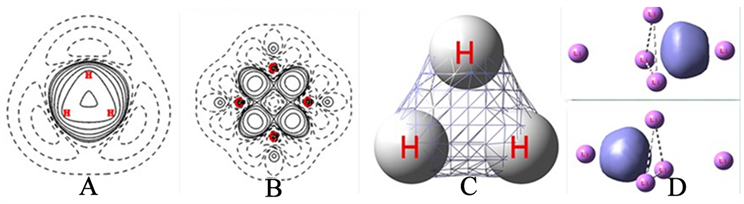Figure 1. (A) $\Delta \rho$ of ${\text{H}}_{{}_{\text{3}}}^{+}$ , (B) $\Delta \rho$ of C4H8, (C) AdNDP of ${\text{H}}_{{}_{\text{3}}}^{+}$ , (D) AdNDP of $L{i}_{5}^{+}$

B2H6的MO7，见图2(B)。这里中间的青色表达了四中心原子对电子的吸引，所以它们是同一个相位的。同时也表明了这个多中心键体系与另外四个通常的B-H键是相互排斥的，它们是反相位的。

B2H6的MO5，见图2(C)。它明确地表明这个四中心是由两个三中心键组成的，而且这两个三中心键是相位是完全相反的，是相互排斥的，这里表达了两个3c2e键的键电子的排斥。和分子中所有键一样，对于邻近的键，有的MO会表明它们的MO是相位相符在一起的，因为这一方的原子也会吸引对方的电子，表明它们之间是有引力的。另有的MO会表明它们的MO是反相位在一起的，因为这一方的键上的配对电子会排斥对方的键上配对电子，表明它们之间是相互排斥的。分子的结构正是这吸引和排斥的平衡，对立的统一。

MO5表明两个三中心键是反相位、独立存在的，一个三中心键共享两个电子，它们之间像通常两个邻近键那样有相互作用，既有同相位MO7，又有反相位MO5。

3.3. 三硼烷B3H9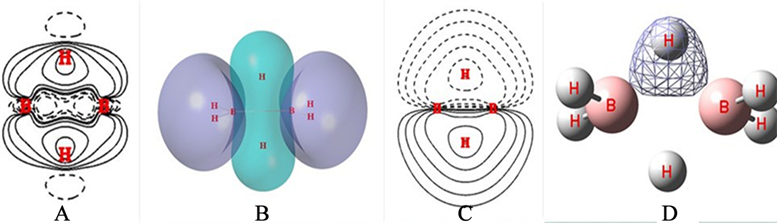Figure 2. (A) $\Delta \rho$ of B2H6, (B) MO7 of B2H6, (C) MO5 of B2H6, (D) AdNDP of B2H6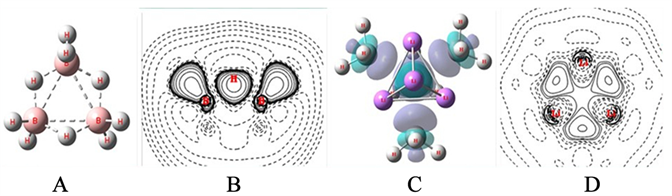Figure 3. (A) Structure of B3H9, (B) $\Delta \rho$ of B3H9, (C) $\Delta \rho$ of Li4(CH3)4, (D) Section contours of $\Delta \rho$ of Li4(CH3)4

3.4. 四聚甲基锂Li(CH3)4

4. 再谈多中心键的概念

4.1. 为什么σ-超共轭本身不构成多中心键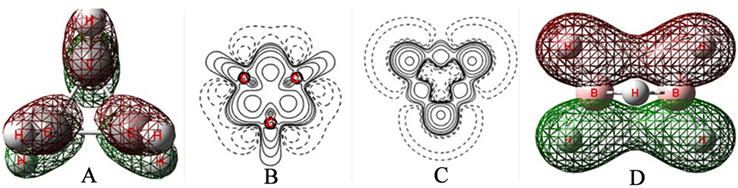Figure 4. (A) σ-hyper conjugative of C3H6, (B) $\Delta \rho$ of C3H6, (C) Section of $\Delta \rho$ of C3H6, (D) σ-hyper conjugative of B2H6

CH2、BH2这样的分子片部件，还可以与共轭π键对叠起来成为σ,π-超共轭，图5(A)是用MP2方法优化计算的自由基CH2=CH-CH2的MO8图像，它反映了自由基处的CH2与C=C的π键相位相符的σ,π-超共轭形象。由于有超共轭，自由基处的C-C单键由1.49869宿短至1.47873了。

σ-超共轭以及具有σ-芳香性，是键与键之间的关系、效应。而多中心键只是一个单一的键，它本身没有σ-超共轭以及σ-芳香性的问题。

4.2. 为什么苯分子不构成多中心键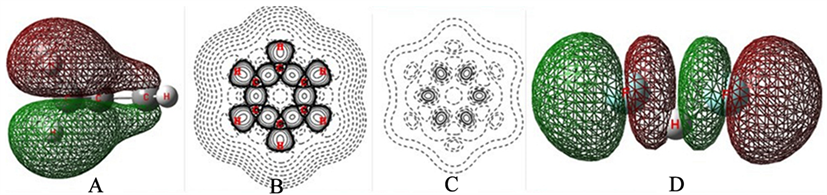Figure 5. (A) σ,π-hyper conjugative of CH2=CH-CH2, (B) σ- $\Delta \rho$ of C6H6, (C) π- $\Delta \rho$ of C6H6, (D) MO10 of (FHF)−1

4.3. 为什么多中心键没有σ和π的内容和形式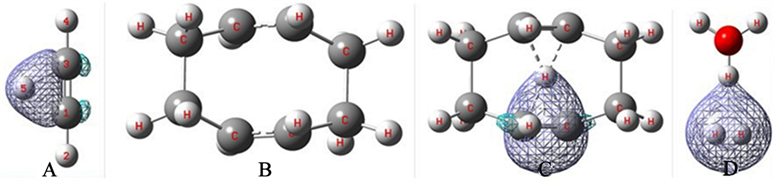Figure 6. (A) AdNDP of HC≡CH…H+, (B) Structure of COD, (C) AdNDP of COD…H+, (D) AdNDP of [H3OH2]+

4.4. 链状分子不构成多中心键

5. 结论

A Quantum Chemistry Study of the Chemical Bond (3)[J]. 物理化学进展, 2018, 07(03): 121-130. https://doi.org/10.12677/JAPC.2018.73015

1. 1. Levine, I.N., 著. 量子化学[M]. 宁世光, 余敬曾, 刘尚长, 译. 北京: 人民教育出版社, 1981.

2. 2. 周光耀. 化学键的量子化学研究(一) [J]. 物理化学进展, 2018, 7(1): 9-18.

3. 3. Frisch, M.J., Trucks, G.W., Schlegel, H.B., Scuseria, G.E., Robb, M.A., Cheeseman, J.R., Scalmani, G., Barone, V., Petersson, G.A., Nakatsuji, H., Li, X., Caricato, M., Marenich, A.V., Bloino, J., Janesko, B.G., Gomperts, R., Mennucci, B., Hratchian, H.P., Ortiz, J.V., Izmaylov, A.F., Sonnenberg, J.L., Williams-Young, D., Ding, F., Lip-parini, F., Egidi, F., Goings, J., Peng, B., Petrone, A., Henderson, T., Ranasinghe, D., Zakrzewski, V.G., Gao, J., Rega, N., Zheng, G., Liang, W., Hada, M., Ehara, M., Toyota, K., Fukuda, R., Hasegawa, J., Ishida, M., Nakajima, T., Honda, Y., Kitao, O., Nakai, H., Vreven, T., Throssell, K., Montgomery, Jr., J.A., Peralta, J.E., Ogliaro, F., Bearpark, M.J., Heyd, J.J., Brothers, E.N., Kudin, K.N., Staroverov, V.N., Keith, T.A., Kobayashi, R., Normand, J., Raghavachari, K., Rendell, A.P., Burant, J.C., Iyengar, S.S., Tomasi, J., Cossi, M., Millam, J.M., Klene, M., Adamo, C., Cammi, R., Ochterski, J.W., Martin, R.L., Morokuma, K., Farkas, O., Foresman, J.B. and Fox, D.J. (2016) Gaussian 16, Revision A. 03. Gaussian, Inc., Wallingford CT.

4. 4. Lu, T. and Chen, F.W. (2012) Multiwfn: A Multifunctional Wavefunction Analyzer. Journal of Computational Chemistry, 33, 580-592. https://doi.org/10.1002/jcc.22885

5. 5. 王紫薇. 高压下铍–氢和氯–氢化合物的结构与物性[D]: [博士学位论文]. 长春: 吉林大学, 2015.

6. 6. Chai, J.-D. and Head-Gordon, M. (2008) Long-Range Corrected Hybrid Density Functionals with Damped Atom-Atom Dispersion Corrections. Physical Chemistry Chemical Physics, 10, 6615-6620. https://doi.org/10.1039/b810189b

7. 7. Zubarev, D.Y. and Boldyrev, A.I. (2008) Developing Paradigms of Chemical Bonding: Adaptive Natural Density Partitioning. Physical Chemistry Chemical Physics: PCCP, 10, 5207-5217. https://doi.org/10.1039/b804083d

8. 8. Grimme, S. (2006) Semiempirical Hybrid Density Functional with Perturbative Sec-ond-Order Correlation. The Journal of Chemical Physics, 124, Article ID: 034108. https://doi.org/10.1063/1.2148954

9. 9. Weht, R.O., Kohanoff, J., Estrin, D.A. and Chakravarty, C. (1998) An Ab Initio Path Integral Monte Carlo Simulation Method for Molecules and Clusters: Application to Li4 and Li5+. Journal of Chemical Physics, 108, 8848-8858. https://doi.org/10.1063/1.476331

10. 10. King, RB. (2003) Metal Cluster Topology. 21. Sigma Aromaticity in Triangular Metal Carbonyl Clusters. Inorganica Chimica Acta, 350, 126-130. https://doi.org/10.1016/S0020-1693(02)01501-3

11. 11. 阎世英, 马美仲, 朱正和. B2 H6分子的几何构型[J]. 物理学报, 2005(54): 3106-3110.

12. 12. 武海顺, 潘道暟, 周伟良. B3H7和B3H9分子中多中心键的研究[J]. 南京理工大学学报, 1994(3): 1-6.

13. 13. 刘亨, 杜奇石, 孙玉彬, 王树青. 四聚甲基锂Li4(CH3)4的电子结构的量子化学研究[J]. 天津师范大学学报(自然科学版), 2004, 24(2): 9-11.

14. 14. Cremer, D. (1988) Pros and Cons of σ-Aromaticity. Tetrahedron, 44, 7427-7457. https://doi.org/10.1016/S0040-4020(01)86238-4

15. 15. Dewar, M.J.S. and Pettit, R. (1954) A Molecular-Orbital Theory of Or-ganic Chemistry. Part VII. The Additivity of Bond Energies in Unconjugated Systems. Journal of the Chemical Society, 74, 1625-1634. https://doi.org/10.1039/jr9540001625

16. 16. 陈姝璇, 陈秀梅, 吕鑫. 乙硼烷及其同系物的σ芳香性[J]. 厦门大学学报(自然科学版), 2016, 55(3): 305-308.